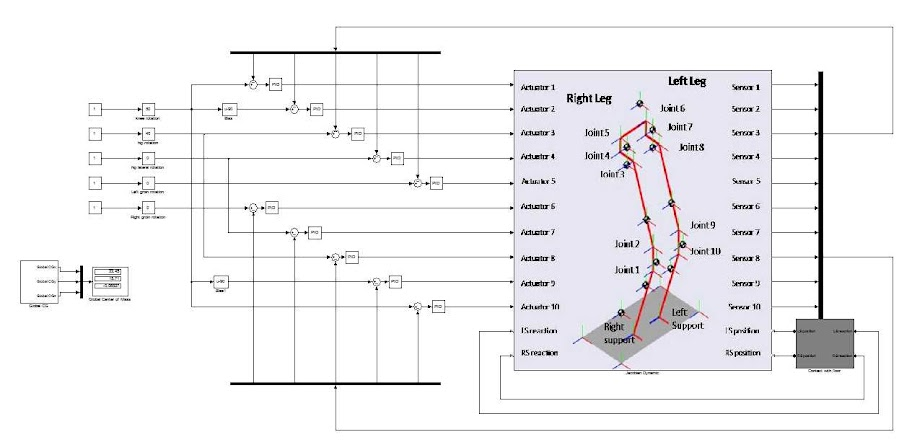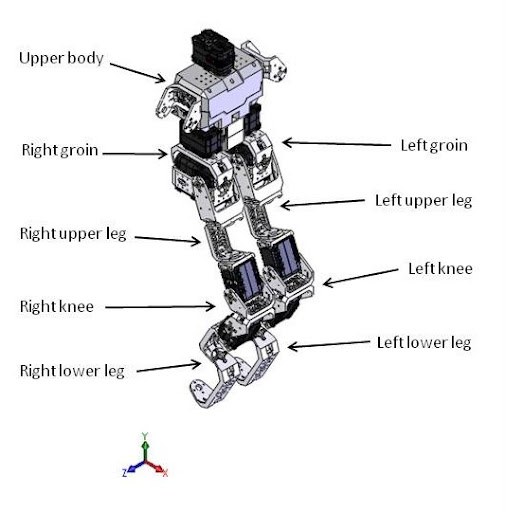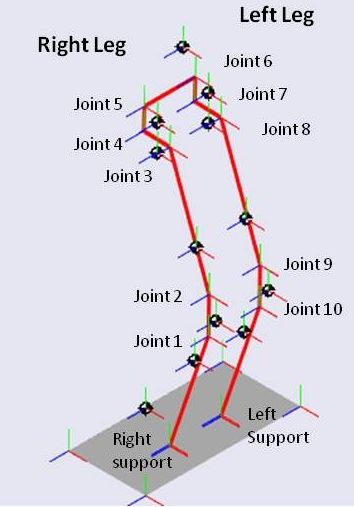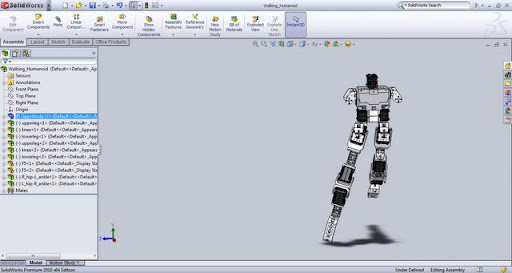## Author Archive

### EZPhysics Model Identification

May 13, 2011

Following the methodology addressed in the last post to identify the dynamic in order to stabilize the humanoid like a triple inverted pendulum, we performed a new approach based in an identification (ARX model) with a greater order and a truncation to the desired order.

The results were very good, and its dynamic responses virtually identical to EZPhysics responses. See the detailed methodology and results here.

The follow steps is tune an LQR in the identified model to then apply it to model EPZhysics.

### Model Identification

April 19, 2011

The first step to control the humanoid motion its own stabilization. To perform this stabilization, we pretend to implement a discrete LQR (Linear Quadratic Regulator), which implement a gain matrix in a control close loop. The control system will operate at a sampling rate that is limited by the frequency of communication between the controller (PC / matlab) and robot (real or virtual). In the first approach, we consider that the contact point between the feet and the floor is a rotational joint without sensors or actuators. Thus, the point of contact with the ground only has 1 DoF, to control the stability of the robot is required only the pitch signal from the IMU.Applied Methodology:
In our approach we will identify the model like a 3 link suspended pendulum to achieved the dynamic of the real model (3 link inverted pendulum) rotating the poles (characteristic of the dynamic system). After to obtain the dynamic of the 3 link inverted pendulum, should be possible to implement a LQR to stabilise the system.

For more detailed information about the methodology to implement the LQR in a 3 link inverted pendulum from the suspended model, see the report here.

Simulations and results:
The simulations was implemented with a frame rate of 200Hz (0.005s).

In our approach we try to obtain the model through the MatLab Identification Toolbox (we use an ARX model) and with the classic dynamic rules.

The detailed information about the obtain data, the methods and first obtain models for the real robot and the EZPhisics, see the report here.

### Implementation of a LQR in a 3 inverted pendulum (simulink model))

April 19, 2011

To implement the humanoid motion, the first step that need to achieved is its stabilization.
Stabilization is to keep quiet in a humanoid in a certain position.
In first approach, we will considerer that robot has the two legs parallels and the foot connect with the floor with 1DoF.
Thus, the control stabilization of the jacobian is similar like the stabilization of a 3 inverted pendulum under-actuated.
Before the tests with the real or the EZphisics model we perform the first tests with a MatLab/Simulink model, build with the SimMechanics blocks.
For detailed information about the construction of the 3 inverted link model and the implementations of the LQR in MatLab/Simulink see the report here.

Simulations results (continuous LQR):
We performed simulations of the system under vertical stabilization, corresponding to a period of 5 seconds. To analyze the robustness of the LQR we implemented an abrupt perturbation of 1N.m in the torque (along 1 second) of the 2nd actuator in t=1s.

Results for the follow  Ricatti equation parameters:
– Q = 0.1xI6
– R = 1xI2

Results for the follow  Ricatti equation parameters:
– Q = 10xI6
– R = 1xI2

Simulations results (discrete LQR):
Considering that the humanoid will be controlled through a PC with a sampling rate of 100Hz, it is necessary to design a digital controller for this condition.

To analyze the robustness of the LQR we implemented an abrupt perturbation of 0.25N.m in the torque (along 0.2 second) of the 2nd actuator in t=1s.

Results for the follow  Ricatti equation parameters:
– Q = 10xI6
– R = 1xI2

March 27, 2010

The Simulink model of Jacobian has a close loop for each joint with a PID controller to keep all joints in a predefined position, another close loop to simulate the contact with the ground and a block to estimate the global center of mass of Jacobian.
The simulink model was built with SimMechanics blocks where each body block has the values of mass, center of mass and inertia (from here) and joints coordinates acquired from 3D model.
In this model is possible to simulate movements of joints but, at a moment, the knee and hip rotations is equals to the both legs.The next steps are the control of equilibrium of Jacobian. First we will control the equilibrium with two legs on the ground and later with only one.

To finalize the equilibrium control of Jacobian, we will do it when the humanoid stand down and up, bending the knees.

### Mass proprieties of jacobian – Dynamixels in center position

March 27, 2010

In this study, we repeted the  process to acquire the mass proprieties of Jacobian with the all dynamixels in center value. This pose is better than the another one (in approximate equilibrium point) because we has better information about the rotation angle of joints.We use the Mass Properties tools of Solid Works to get the proprieties of each individual body. In the first stage we performed a full body analyze, and later we suppress 10 assemblies (assembly of parts for each individual body) for each analysis to get proprieties of individual body parts.

The properties of this study were defined with all dynamixels in center value and should be available here.

These are the values that we used in the MatLab/Simulink model

### Mass Properties of Jacobian (in equilibrium point)

March 25, 2010

The Kangaroo (a.k.a Jakobian1) has a multi-body structure with 11 bodies and 10 joints.In this study, we used the Mass Properties tools of Solid Works to get the proprieties of each individual body. In the first stage we performed a full body analyze, and later we suppress 10 assemblies (assembly of parts for each individual body) for each analysis to get proprieties of individual body parts.

The properties of this study were defined with a robot pose approximated at the equilibrium point and should be available here.

We will use this values to build a model in MatLab/Simulink.

### Jacobian model in SimMechanics (MatLab/Simulink)

March 5, 2010

We did a first approach to build the jacobian dynamic model in MatLab 2009b / Simulink using the blocks of SimMechanics Toolbox.

In this first approach, we used he properties of the structure and body parts with a robot pose approximated at the equilibrium point.

The SimMechanics blocks needs some parameters (center of mass and inertia matrix of each body part and joint coordinates) that are obtained in report “Mass Properties of Jacobian – equilibrium point

To view the simulation, download the model, start the Matlab 2009b (the model could not open in prior versions), open the Simulink model and click in “Start” and view the structure in the machine for model.### 3D Model of ‘Jacobian1’ the Kangaroo Robot

February 23, 2010

Using the 3D models of components from the Bioloid robot kit available from http://humanoids.dem.ist.utl.pt, we built in SolidWorks the 3D model of our Kangaroo Robot (download SolidWorks Model).

The objective is to calculate the mechanical proprieties (inertial moment, weight, center-of-mass) of each part of robot’s body (torso, hip, upper leg, etc…) and also create a WRL model that can be animated by MatLab/Simulink.

The Kangaroo (a.k.a Jakobian1) has 11 different sub-assemblies/parts (torso and two for each hip, upper and lower leg and knee for each leg). Mechanical properties need to be calculated for each in order to model the structure and be able to control its motion.

By enabling axis constraints, we can even mold the robot into some inspirational poses.